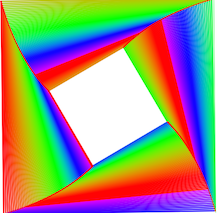# Iteration in the Complex Plane

The cover story of the April 2014 issue of Mathematics Teacher is on “Iteration in the Complex Plane”, by Robin S. O’Dell. It sounds like pretty advanced mathematics, but is surprisingly accessible with The Geometer’s Sketchpad.

It’s all based on the surprising principle that you can multiply two complex numbers very easily if you express them as magnitude (sometimes called the modulus) and polar angle (sometimes called the argument). To multiply two complex numbers expressed in this form, all we need to do is to multiply the magnitudes and add the angles.

The iterations in the article are based on starting with one complex number (the seed) and multiplying repeatedly by another complex number (the multiplier). In the sketch below, you can drag S to change the seed, and you can edit the values of rM and θM to change the modulus and angle of the multiplier. (By controlling the multiplier numerically, you can make very fine adjustments to its value.) Change depth to determine how many additional times the multiplication is performed.

Begin your experimentation by changing the values as described in the sketch itself:

As a second exercise, you can produce an image similar to the first one in the article (on pp.592–593) by setting rM to 0.988, θM to 90.4°, and depth to 100. By changing these values, you can produce each of the regular polygons, rotated polygons, and star polygons described in the article.

Even more striking results ensue when you add color, using the depth of each segment to determine its color. Press the Next button to go to the second page of the sketch, and try the experiment described there.

The colors parameter determines how many colors of the spectrum are used before returning to the original color, so setting colors to 3 results in a design that alternates among three colors. When colors is not a whole number (for instance, 4.05), the fifth segment will have a very slightly different color from the first. By choosing colors that interact with the geometric nature of the complex design, you can produce some very lovely effects, effects that rival the color work shown in Figure 8 in the article.